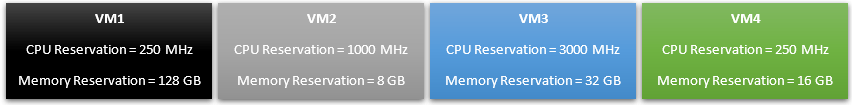# vSphere HA Slots Policy DemystifiedPosted by

### Introduction:

When you choose Slot policy, the failover capacity is ensured using a Slot based approach. In vSphere release 6.5 and above  you may choose a fixed slot size as well

#### What is a Slot and Slot Size ?

If we assume an ESXi to be a car parking lot, A Slot is a space where a VM (VM is the car here) can parked and Slot Size is the dimension of individual parking spot.

Lets say you have a task at hand of converting a huge arear to a Car park with fixed dimensions of an individual parking slots. How will you do it?

One way to do this to find the Length of the longest vehicle (LV) and the Width of the widest vehicle (WV) that can visit this car park. Hence, dividing the whole area into slots of size LV * WV

Unfortunately  this is not what vSphere does. vSphere makes this problem one dimensional

### Let’s look at an Example:

HA slot calculation algorithm sets aside about 20% of total CPU and Memory resources  for VMkernel and provides remaining for the VMs to use. This 20% is hard coded. I was unable to find a VMware document stating this. However,  the disparity noticed in HA Run time information and the calculations without considering the 20% overhead proves the hypothesis.

You may test it for one of your HA clusters.

#### CPU Resources:

Let’s assume Number of hosts in the cluster (NHC) = 4

Let’s assume Number of CPU on each host (NCPH) = 4

Let’s assume Number of Cores per socket (NCPS)  = 8

Let’s assume CPU Speed (CS) = 2.2 GHz

Total CPU resources available per host without HT(CRPHWOHT) = NCPH * NCPS * CS = 4 * 8 * 2.2 = 70.4 GHz

Total CPU resources available per host with HT(CRPHWHT) = CRPHWOHT * 2 = 70.4 * 2 = 140.8 GHz

Total CPU resources available in cluster (CRAC) = CRPHWHT * NHC = 140.8 * 4 = 563.2 GHz

#### Memory Resources:

Let’s assume amount of Memory on each host (MEH) = 512 GB

Total amount of Memory in cluster (TMC) = MEH * NHC = 512 * 4 = 2,048 GB

#### Reservations:

Let’s assume we have 4 VMs with reservations

Maximum CPU reservation configured (MCRC) = 3000 Mhz (Check Blue VM)

Maximum Memory reservation configured (MMRC) = 128 GB (Check Black VM)

#### Total CPU and Memory available for VMs:

Total CPU available for VMs (TCAV) = CRAC – (.2 * CRAC ) = 563.2 – 112.64 =  450.56 GHz

Total Memory available for VMs (TMAV) = TMC – (.2 * TMC ) = 2048 – 409.6 = 1638.4 GB

#### Therefore:

Total Slots in the cluster based on CPU (TSBC) = TCAV/MCRC = 450.56/3 = 150.1867

Total Slots in the cluster based on Memory (TSBM) = TMAV/MMRC =  1638.4/128 = 12.8

Total Slots in the cluster (TSC) = Round down the Minimum of (TSBC & TSBM) to 0 decimal = 12

#### Summary:

From the example above we see that the cluster will only allow to power on 12 VMs when HA admission control is ON with default configuration. Hence, it is imperative to configure %failover resources in such case to utilize the cluster capacity effectively.

The recommendation would be to follow the HA hard coded overhead (20%) when doing manual calculations as well.  In the above example TCAV and TMAV are the effective CPU and Memory  resources available for VMs.

vSphere HA uses the actual reservations of the virtual machines. If a virtual machine does not have reservations, meaning that the reservation is 0, a default of 0MB memory and 32MHz CPU is applied.

### Attachment:

The file attached below is the excel implementation of above calculation. Feel fee to download and use for your design.

Comment in case you notice any issues with it.

## One comment

1.MxAdm!n says:

Very important information your shared in this post and unveiled the 20% compute reservation mystery.

This site uses Akismet to reduce spam. Learn how your comment data is processed.